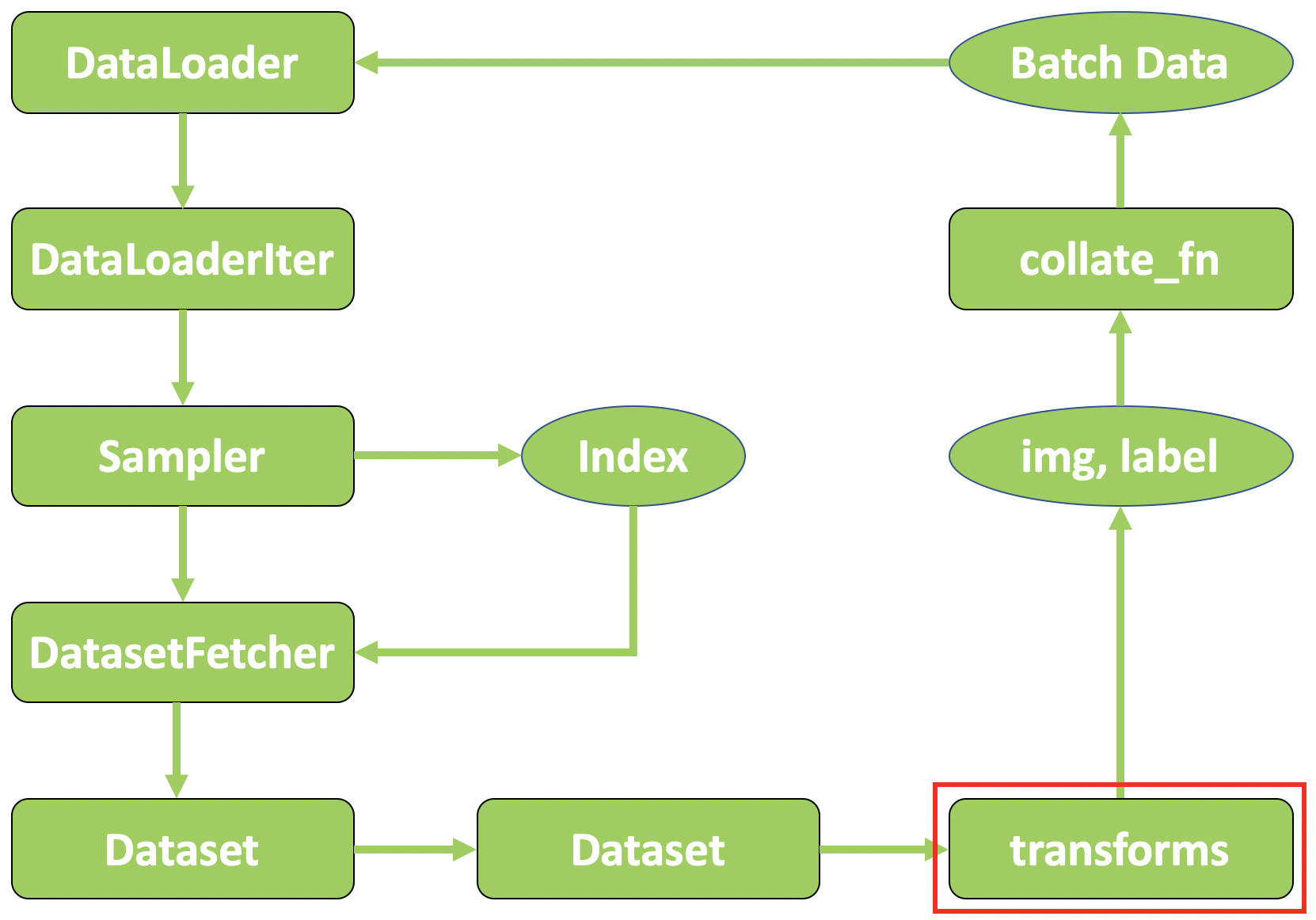# PyTorch 07：数据预处理 transforms 模块机制

## PyTorch 学习笔记

Posted by YEY on December 11, 2020

# Lecture 07 数据预处理 transforms 模块机制

## 1. 数据预处理 transforms 机制

#### torchvision：计算机视觉工具包

• torchvision.transforms：常用的图像预处理方法。
• torchvision.datasets：常用数据集的 dataset 实现，MNISTCIFAR-10ImageNet 等。
• torchvision.model：常用的模型预训练，AlexNetVGGResNetGoogLeNet 等。

#### torchvision.transforms：常用的图像预处理方法

• 数据中心化
• 数据标准化
• 缩放
• 裁剪
• 旋转
• 翻转
• 填充
• 噪声添加
• 灰度变换
• 线性变换
• 仿射变换
• 亮度、饱和度及对比度变换#### 例子：人民币二分类中的 transforms

1
2
3
4
5
6
7
8
9
10
11
12
13
14
15
16
17
18
19
20
21
22
23
24
25
26
27
28
29
30
31
32
33
34
35
36
37
38
39
40
41
42
43
44
45
46
47
48
49
50
51
52
53
54
55
56
57
58
59
60
61
62
63
64
import os
import random
import numpy as np
import torch
import torch.nn as nn
import torchvision.transforms as transforms
import torch.optim as optim
from matplotlib import pyplot as plt
from model.lenet import LeNet
from tools.my_dataset import RMBDataset

def set_seed(seed=1):
random.seed(seed)
np.random.seed(seed)
torch.manual_seed(seed)
torch.cuda.manual_seed(seed)

set_seed()  # 设置随机种子
rmb_label = {"1": 0, "100": 1}

# 参数设置
MAX_EPOCH = 10
BATCH_SIZE = 16
LR = 0.01
log_interval = 10
val_interval = 1

# ========================= step 1/5 数据 ===============================
split_dir = os.path.join("..", "..", "data", "rmb_split")
train_dir = os.path.join(split_dir, "train")
valid_dir = os.path.join(split_dir, "valid")

norm_mean = [0.485, 0.456, 0.406]
norm_std = [0.229, 0.224, 0.225]

# Compose 会将一系列的 transforms 操作进行组合包装，按顺序执行
train_transform = transforms.Compose([
# 将图像缩放到 32*32 大小
transforms.Resize((32, 32)),
# 对图像进行随机裁剪（数据增强）
# 将图片转成张量形式，并进行归一化操作，把像素值区间从 [0, 255] 归一化到 [0, 1]
transforms.ToTensor(),
# 数据标准化，均值为 0，标准差为 1：output = (input - mean) / std
transforms.Normalize(norm_mean, norm_std),
])

# 注意：测试数据不需要进行数据增强
valid_transform = transforms.Compose([
transforms.Resize((32, 32)),
transforms.ToTensor(),
transforms.Normalize(norm_mean, norm_std),
])

# 构建 MyDataset 实例
train_data = RMBDataset(data_dir=train_dir, transform=train_transform)
valid_data = RMBDataset(data_dir=valid_dir, transform=valid_transform)



PyTorch 中的数据预处理流程图## 2. 数据标准化：transforms.Normalize

#### transforms.Normalize

1
2
3
4
5
transforms.Normalize(
mean,
std,
inplace=False
)


• mean：各通道的均值。
• std：各通道的标准差。
• inplace：是否原地操作。

output = (input - mean) / std

## 3. 总结本作品采用知识共享署名-非商业性使用-相同方式共享 4.0 国际许可协议进行许可。 欢迎转载，并请注明来自：YEY 的博客 同时保持文章内容的完整和以上声明信息！